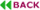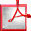doi: 10.7873/DATE.2015.0731

# ECRIPSE: An Efficient Method for Calculating RTN-Induced Failure Probability of an SRAM Cell

#### Graduate School of Informatics, Kyoto University, Yoshida-honmachi, Sakyo-ku, Kyoto, Japan.

paper@easter.kuee.kyoto-u.ac.jp

## ABSTRACT

Failure rate degradation of an SRAM cell due to random telegraph noise (RTN) is calculated for the first time. ECRIPSE, an efficient method for calculating the RTNinduced failure probability of an SRAM cell, has been developed to exhaustively cover a large number of possible bias-voltage combinations on which RTN statistics strongly depend. In order to shorten computational time, the Monte Carlo calculation of a single gate-bias condition is accelerated by incorporating two techniques: 1) construction of an optimal importance sampling using particles that move about the “important” regions in a variability space, and 2) a classifier that quickly judges whether the random samples are in failure regions or not. We show that the proposed method achieves at least 15.6× speed-up over the state-of-the-art method. We then integrate an RTN model to modulate failure probability. In our experiment, RTN worsens failure probability by six times than that calculated without the effect of RTN.Full Text (PDF)